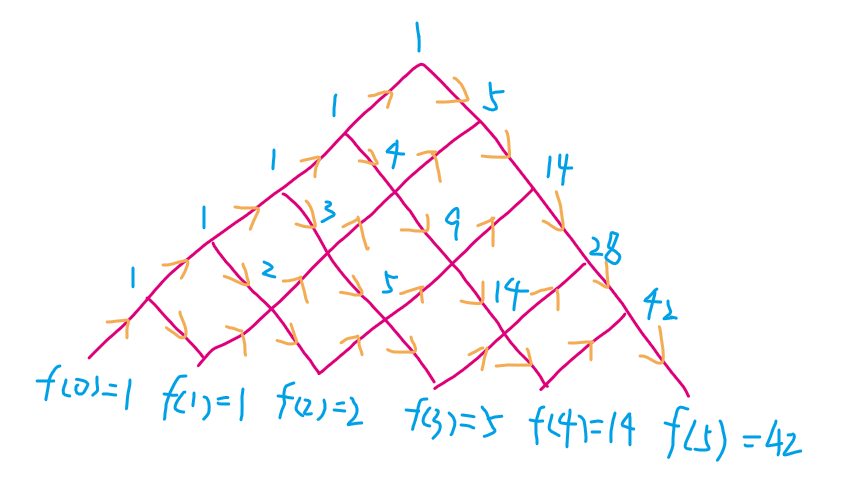*这是本博客第一篇使用 $LaTeX$ 语法的文章，如有错误还请见谅。

1,1,2,5,14,42,132,429,1430,4862,16796,58786,208012,742900, ...


1730年我国清朝时期的明安图（蒙古人）比 Catalan 更早使用了 Catalan 数，见《割圜密率捷法》。

## 公式

$f(n) = \sum_{i=0}^{n-1}f(i) \times f(n-i-1)$

$f(n) = \frac{f(n-1) \times (4 \times n - 2)}{n+1}$

$f(n)= \frac{C_{2n}^{n}}{n+1}$

$f(n)=C_{2n}^{n}-C_{2n}^{n-1}$

## 应用

1. 连乘括号化
2. 出栈次序
3. 凸多边形三角形划分
4. 给定节点组成二叉树
5. $n$ 对括号正确匹配

### 二叉树的计数

#### 解题思路

$f(n)=\sum_{i=0}^{n-1}f(i) \times f(n-i-1)$

### AB排列问题

#### 问题描述

$n=1$ 时：AB，$f(1)=1$
$n=2$ 时：AABB ABAB，$f(2)=2$
$n=3$ 时：AAABBB AABBAB ABAABB ABABAB，$f(3)=5$

#### 解题思路## 参考资料

《信息学奥赛之数学一本通》 林厚从

【学习笔记】卡特兰数

0%